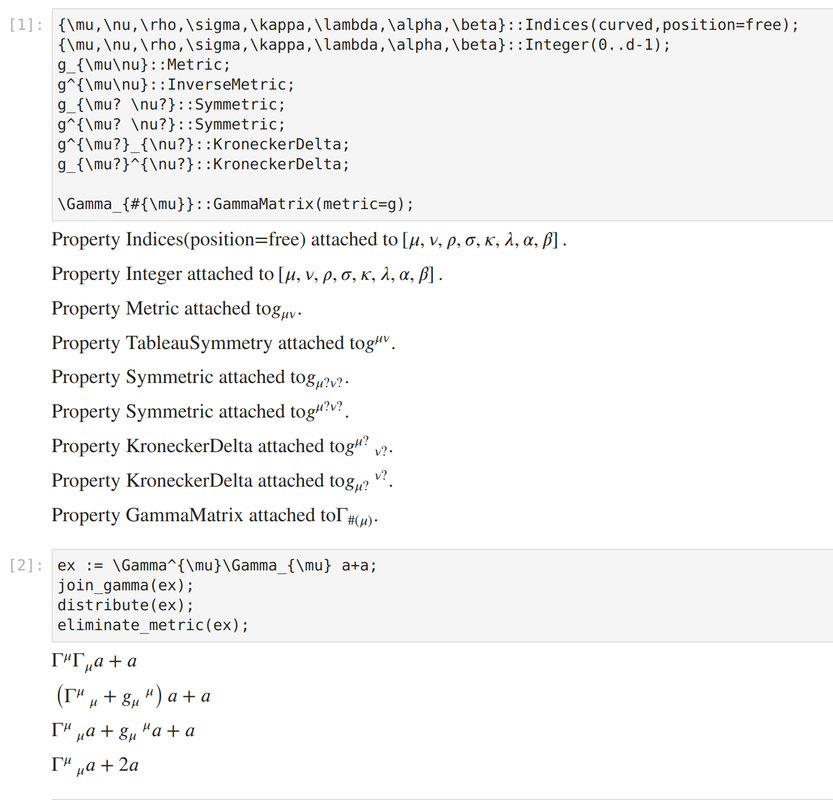# Issue with eliminate metric

+1 vote

Hello! I'm new to Cadabra and recently I've noticed a strange behaviour of the function eliminate_metric.

Here is a simple code demonstrating this:

{\mu,\nu,\rho,\sigma,\kappa,\lambda,\alpha,\beta}::Indices(curved,position=free);
{\mu,\nu,\rho,\sigma,\kappa,\lambda,\alpha,\beta}::Integer(0..d-1);
g_{\mu\nu}::Metric;
g^{\mu\nu}::InverseMetric;
g_{\mu? \nu?}::Symmetric;
g^{\mu? \nu?}::Symmetric;
g^{\mu?}_{\nu?}::KroneckerDelta;
g_{\mu?}^{\nu?}::KroneckerDelta;

\Gamma_{#{\mu}}::GammaMatrix(metric=g);

ex := \Gamma^{\mu}\Gamma_{\mu} a+a;
join_gamma(ex);
distribute(ex);
eliminate_metric(ex);

What this code supposed to do is to perform simple gamma matrix calculations, paying no attention to a random variable "a". As a result, there should be a term "d * a". But, instead, I yield thisThe function eliminatemetric somehow dropped away g{\mu}^{\mu}.

Is it just my bad code or a real bug? I understand that the fix is to insert eliminate_kronecker, but I can't be sure that such a problem won't appear in another unexpected place.

If you want to use metrics to raise/lower your indices, then you cannot declare them as position=free. The latter means that the position of indices (subscript/superscript) has no meaning and can be changed arbitrarily, without factors of the metric. But worse, it means that all your patterns collapse to a single one, because upper indices will match lower indices and vice versa.
If you change your first line to contain position=fixed then things work as expected. The eliminate_metric will then not do anything (since there is no metric in your expression), but eliminate_kronecker will replace the $g_{\mu}{}^{\mu}$ with $d$. A further canonicalise will remove the $\Gamma^{\mu}{}_{\mu}$.